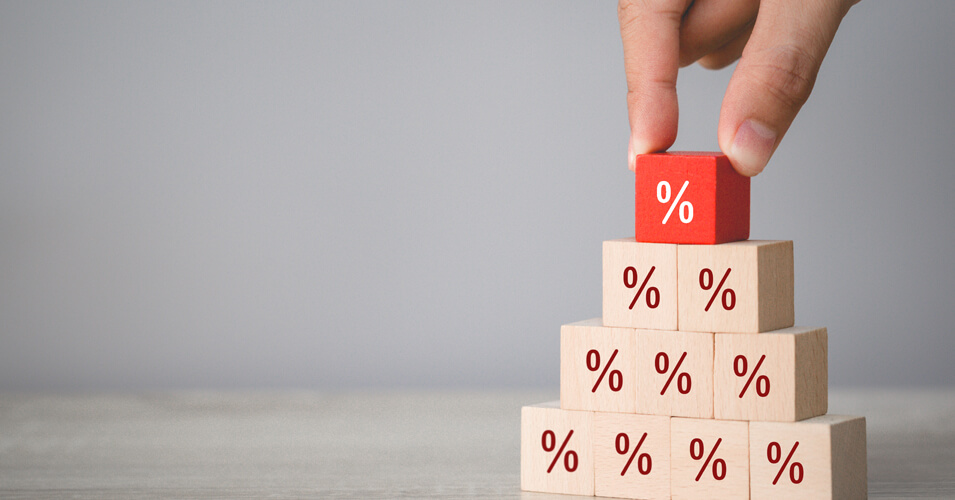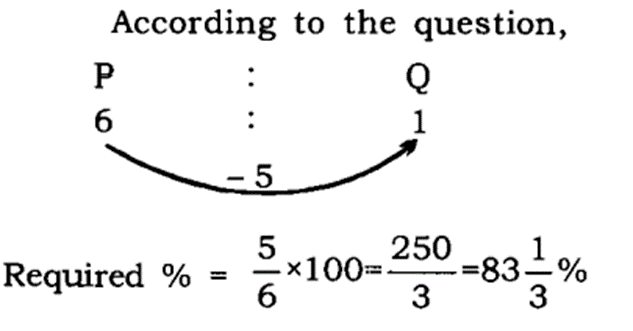• Save

# Percentage Aptitude Questions for Competitive ExamsPercentage is a way of expressing a ratio in a mathematics subject. Also, percentage is very important in all competitive examinations, because other topics of mathematics also depend on percentage. Percent means per hundred (% = 1/100). Today in this article, I am providing percentage aptitude questions for all those students, who are preparing day-night for successful results in competitive exams. Important questions given here which are often asked in competitive exams. These questions are useful for all exams like- SSC CGL, SSC CHSL, IBPS PO, IBPS CLERK, POLICE, etc. So read them carefully -

## Important Questions Based on Percentage Aptitude Questions

Q :

A man spends a part of his monthly income and saves the rest. The ratio of his expenditure to the saving is 61 : 6. If his monthly income is Rs .8710, the amount of his monthly saving is

(A) रु. 870

(B) रु. 980

(C) रु. 780

(D) रु. 690

Q :

The difference between the value of the number increased by 20 % and the value of the number decreased by 25 % is 36. Find the number.

(A) 720

(B) 80

(C) 7.2

(D) 0.8

Q :

In a test a student got 30 % marks and failed by 25 marks. In the same test another student got 40 % marks and secured 25 % marks more than the essential minimum pass marks. The maximum pass marks for the test were?

(A) 500

(B) 580

(C) 400

(D) 480

Q :

Two numbers are 90 % and 75 % lesser than a third number. By what % should the first number be increased so that it becomes equal to the second number?

(A) 150

(B) 100

(C) 250

(D) 200

Q :

If X is 20% less than Y, then find the value of $${Y-X\over Y}$$ and $${X\over X-Y }$$

(A) $${3\over5},-{3\over5}$$

(B) $${1\over5},-4$$

(C) $$5,-{1\over4}$$

(D) $${2\over5},-{5\over2}$$

Q :

The price of sugar is increased by 20 %. If the expenditure on sugar has to be kept the same as earlier, the ratio between the reduction in consumption and the original consumption is:

(A) 1 : 6

(B) 1 : 5

(C) 1 : 3

(D) 1 : 4

Q :

A man's annual income has increased by Rs. 5 lakhs but the tax on income that he has to pay has reduced from 12 % to 10 %. He now pays Rs. 10,000 more income tax. What is his increased income (in Rs. lakhs)?

(A) 15

(B) 10

(C) 20

(D) 25

Q :

25% of 120 + 40% of 380 = ? of 637

(A) $${4\over7}$$

(B) $${2\over7}$$

(C) $${3\over7}$$

(D) $${1\over7}$$

Q :

P is six times as large as Q. The percentage that Q is less than P, is:

(A) $$63{1\over3}\%$$

(B) 50%

(C) $$83{1\over3}\%$$

(D) 70%

Explanation :Q :

40 % are the passing marks. A student gets 250 marks yet fails by 38 marks. What are the maximum marks?

(A) 800

(B) 840

(C) 720

(D) 750